Car and cyclist

Cyclist started from town at speed 18 km/h. After 1 hour 30 min started the car from town and caught up with the cyclist in 50 minutes. How fast was a car?

Result

v =  50.4 km/h

Solution:

18*(1+30/60+50/60)=v*50/60

50v = 2520

v = 2525 = 50.4

Calculated by our simple equation calculator.

Leave us a comment of this math problem and its solution (i.e. if it is still somewhat unclear...):Be the first to comment!To solve this verbal math problem are needed these knowledge from mathematics:

Do you have a linear equation or system of equations and looking for its solution? Or do you have quadratic equation?

Next similar math problems:

1. Theorem proveWe want to prove the sentence: If the natural number n is divisible by six, then n is divisible by three. From what assumption we started?
2. Double-track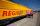On double-track express met with a freight train. Express travel at speed 72 km/h, a freight train at speed 36 km/h. After what time period will be from 9 km apart?
3. Cruise liner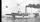Cruise liner runs along the river between points A and B. The journey downstream takes 40 minutes 1 hour upriver. The flow rate of the river is 3 km/h. What is the speed of cruise liner?
4. Klara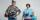Klara and Jitka went on a hiking trip at 13 o'clock at speed 5km/h. At 14 o'clock, Tomas ride on the bike at an average speed of 28 km/h. How many hours and at what distance from the beginning of the road Tomáš caught the two girls?
5. Bob and Bobek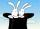At 10h 20min runs Bobek from hat at average speed 12 km/h. At what time must run out Bob at speed 18 km/h to catch him 9 kilometers from the hat?
6. Greg and BillGreg is 18 years old. He is 6 less than 4 times Bill's age. How old is Bill?
7. Simplify 2Simplify expression: 5ab-7+3ba-9
8. GivenGiven 2x =0.125 find the value of x
9. Holidays - on poolChildren's tickets to the swimming pool stands x € for an adult is € 2 more expensive. There was m children in the swimming pool and adults three times less. How many euros make treasurer for pool entry?
10. The ship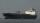The ship went upstream speed of 20 km/h in relation to water. River flows at speed 10 km/h. After half an hour, he stopped and returned downstream to the starting point. How long it took way back when even if downstream speed of ship is 20 km/h in relation
11. CandyPeter had a sachet of candy. He wanted to share with his friends. If he gave them 30 candies, he would have 62 candies. If he gave them 40 candies, he would miss 8 candies. How many friends did Peter have?
12. Simple equationSolve for x: 3(x + 2) = x - 18
13. Six yearsIn six years Jan will be twice as old as he was six years ago. How old is he?
14. Fifth of the numberThe fifth of the number is by 24 less than that number. What is the number?
15. EquationSolve the equation: 1/2-2/8 = 1/10; Write the result as a decimal number.
16. Unknown numberIdentify unknown number which 1/5 is 40 greater than one tenth of that number.
17. Father and sonFather is 48 years old, son 23. When will be the father twice older than a son?